# How multiply matrices in a special way?

All the tutorials I find on the internet all just use keras which do all the math for you. But in a sense what I am trying to do is basically I have whats called a “filter” it is an identity matrix. I also have my “feed” it is another identity matrix except this one is much bigger than the filter (filter is 3x3). So say our feed looks like this

0,0,0,0,0
0,1,1,1,0
0,0,0,1,0
0,0,0,1,0
0,0,0,1,0

and our filter looks like this
1,0,1
0,1,0
1,0,1

Doing this “math” function should return us this matrix

1,2,1,
1,3,2,
0,2,1

This matrix will be called kernel. It should be noted that kernels size is dependent on the filter and feeds size. It was 3x3 here due to the math operation we performed. It is in a sense sliding from left to right multiplying it by each cell in the feed by the filter, the filter is sliding across it multiplying the cells then setting it to the new sum, allocating the 2d array on the rows each slide only allocating to the columns once the filter cant slide anymore because it had reached the end of the feed. The filter cannot go out of the feeds bounds. I am just really stumped on how I am suppose to code this.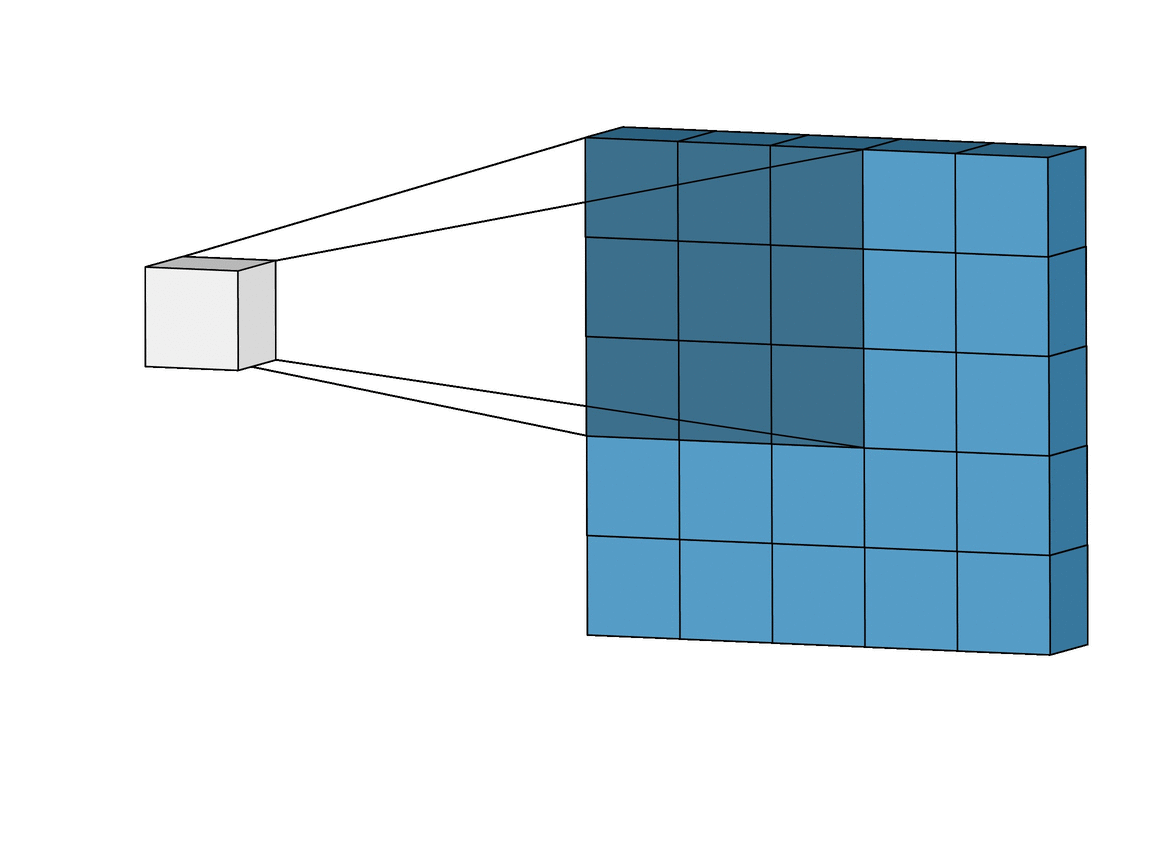2 Likes

So this isn’t an answer but AI things in general in my experience is best answered in Stack Overflow as many programmers are on there and the fact that there is people who post roblox questions there. So my suggestion is also make one on there with this topic if possible

1 Like

im so confused on what you’re trying to do…
the way im understanding it is
we have the matrix here:
0, 0, 0, 0, 0
0, 1, 1, 1, 0
0, 0, 0, 1, 0
0, 0, 0, 1, 0
0, 0, 0, 1, 0

and you have the filter of
1, 0, 1
0, 1, 0
1, 0, 1

so the first 3x3 objects in the matrix
0, 0, 0,
0, 1, 1,
0, 0, 0,
are multiplied by the filter then added together
so the first part of our new matrix is “2”

but that doesnt exactly line up with your results. Can you describe a little more in depth?

Basically we slide the filter across the matrix and then multiple the cells. We then add them all up and insert that into our new allocated matrix 2d array

i see what you mean. I’ll have a go at it

BTW: the main problem is finding that shaded area, the transposing/multiplying part is pretty simple

wait im confused again. How do you get 1 for the result when given the input
0, 0, 0,
0, 1, 1,
0, 0, 0
when multiplied by the filter of 1, and then adding together, it gives you two?

filter is

``````1,0,1
0,1,0
1,0,1
``````

so we can rephrase our feed like

``````0 * 1, 0 * 0, 0 * 1
0 * 0, 1 * 1, 1 * 0
0 * 1, 0 * 0, 0 * 1
``````

It just so happens to be that when we apply this operation then add them all up we get 1.

YES I DID IT OMG IT WAS SO HARD

``````local matrix = {
{0, 0, 0, 0, 0},
{0, 1, 1, 1, 0},
{0, 0, 0, 1, 0},
{0, 0, 0, 1, 0},
{0, 0, 0, 1, 0}
}

local filter = {
{1, 0, 1},
{0, 1, 0},
{1, 0, 1}
}

local function quickmhaffz(matrix, filter)
local oneDimensionMatrix = {}
local newMatrix = {}
for i, v in pairs(matrix) do
for j, x in pairs(v) do
oneDimensionMatrix[(i - 1) * #matrix + j] = x
end
end

local offset = 0
for i, v in pairs(filter) do
newMatrix[i] = {}

for j, x in pairs(v) do
for z = 1, #filter do
local col = ((z - 1) * #filter * 2) - (z - 1) + ((i - 1) * 2)
for t = 1, #filter do
local oneDimensionalIndex = offset + col + t
local n = oneDimensionMatrix[oneDimensionalIndex] * filter[z][t]

if not newMatrix[i][j] then
newMatrix[i][j] = 0
end
newMatrix[i][j] += n
end
end
offset += 1
end
end

return newMatrix
end

print(quickmhaffz(matrix, filter))
``````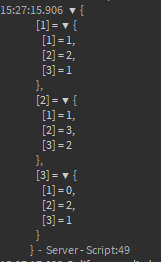my brain hurts this is too much math but there you go, I’ve never really done anything with matrix multiplication but double-check to make sure it works. I’ve only tested it with a filter of only ones, and it checks out. The internet had almost 0 sources on this so this is all my math.

The way it works: convert the matrix into a one-dimensional matrix and then do a embedded for loop through the filter. as the loop goes, add into an offset. (see below) from there do an inbedded for loop again for another 3x3 thing (or filter length) then do math that took a very long time to figure out to get the index we’re looking for in the one-dimensional matrix. multiply that by the position in the filter from the inbedded 3x3 for loop’s x and y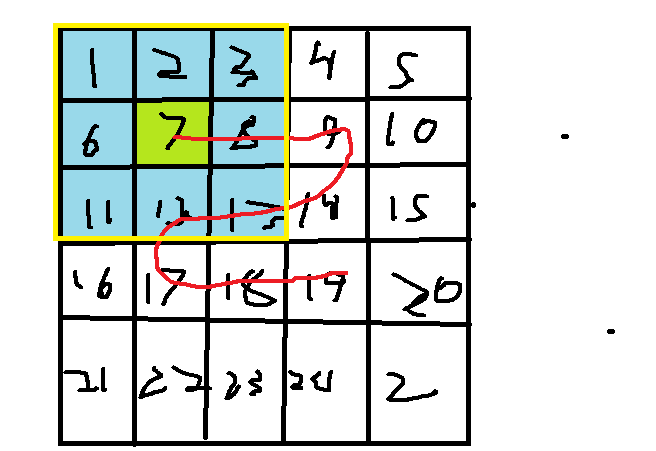this is the way the goes through it, it basically sorts through the inner 3x3 grid and then checks the blocks that surround it. so basically you give it number 1 in the filter and it finds 1, 2, 3, 6, 7, 8, 11, 12, 13 from that. it keeps going around left to right in that inner 3x3 square

It wouldnt work for my feed but for a matrix it would, not sure why (my feed is 30x30)

``````local base = {
{0, 0, 0, 0, 0},
{0, 1, 1, 1, 0},
{0, 0, 0, 1, 0},
{0, 0, 0, 1, 0},
{0, 0, 0, 1, 0},
}

local filter = {
{1, 0, 1},
{0, 1, 0},
{1, 0, 1},
}
-- You can have etc. 5 x 3 filters mapped on 7 x 9 matrices.
local output = {}

-- Filter column & row fitting / check how many placements can be made.
local matrix_fitting_y = (#base - #filter) + 1 -- +1 for first placement & fit in column
local matrix_fitting_x = (#base - #filter) + 1 --  -||- fit in row

-- Construct size of output
for output_tbls = 1, matrix_fitting_y do
table.insert(output, {})
for output_vals = 1, matrix_fitting_x do
table.insert(output[output_tbls], 0)
end
end

-- Per output y and x, we calculate the y, x index of filter toward base & sum it into output.

for mxf_y = 1, matrix_fitting_y do
for mxf_x = 1, matrix_fitting_x do
for y = 1, #filter do
for x = 1, #filter do
local calc = base[(mxf_y + y)-1][(mxf_x + x)-1] * filter[y][x]
if calc >= 1 then
output[mxf_y][mxf_x] += calc
end
end
end
end
end

print(output)
``````

Here you go. It works with a larger base matrix too and will appropriately create the size of the output based on how many filter size iterations can fit in it.

I’m sure you can code golf it for better performance.

Here are some outputs of your 5x5 base with the 3x3 filter: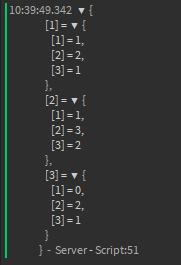Here is one with a 6 x 6 base with the same 3 x 3 filter seen in my script that I sent: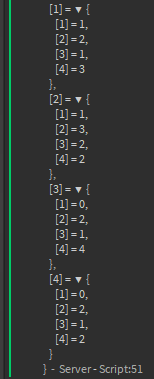You can also create a larger filter and it will automatically calculate the output size for it.

How did I approach this problem?

I first made the same system @Soliform had but then I realized that the base and filter can be larger than that, so it won’t work and the output has to grow along with how many iterations it calculated.

So I realized that you can try to calculate how many times your first row can fit on top of the first row (I first approached it from a 1 dimensional perspective):

``````{0, 1, 0, 1, 0, 1, 0}

{1, 0, 1}
``````

Say your first row is 7 blocks long, how many 3 blocks can fit by jumping by 1 each iteration? I kept my eye on the first index of the filter. How many times can that first index stay inside the 7 blocks without having the entire row go out of bounds? If you take 7 - 3 you get 4 times right? But if you calculate it, you’ll see that you could do it 5 times. This is because the first placement isn’t counted, we’re always adding by 1 from the beginning of our calculation, so add 1. That’s how I got my matrix_fitting. Now if you take a larger filter you’ll see that this logic will now apply to it perfectly.

`matrix_fitting = (#base - #filter) + 1`

Now we can use this fitting to calculate the output. So in this example I’m talking about, (7 - 3) + 1 = 5, so we construct a 5 x 5 output and fill it with empty values.

Now we calculate the possible fittings in the base Y, and loop X inside. X always jumps N amount of times (matrix_fitting times) each Y iteration so the X for loop goes inside Y obviously.

Now inside this base matrix X loop, we can start our filter matrix calculation, looping through X within Y the length of the filter.

So now all we do is make a new fitting, take the position in the base and calculate the y and x on top of it respectively to get the position of where the filters current index is in the base - 1 due to index problem caused by for loops starting at 1.

`if base[(mxf_y + y)-1][(mxf_x + x)-1] * filter[y][x] >= 1 then`

Now we calculate the value of the fittings value that got returned against our filters value which it is currently on and multiplies it. If it returns 1 or greater (which it would if you have 2’s or something), then we can simply take the position of the output and add 1 to it. If the loop finds another 1 in the current frame, it adds another +1 to that very same output index value.

(Btw if you want to check if the filter is greater than the base it’ll be super easy: If the matrix_fitting is below 0)

When the loop is done, we can output the matrix!

EDIT:
Edited the code so you can now have non equal sided matrices like for example a base matrix: 9 x 7 which gets mapped on etc 3 x 5 filters!EDIT V2:
Now if you have values higher than 1 in either the base or the filter, the output will appropriately calculate the sum.